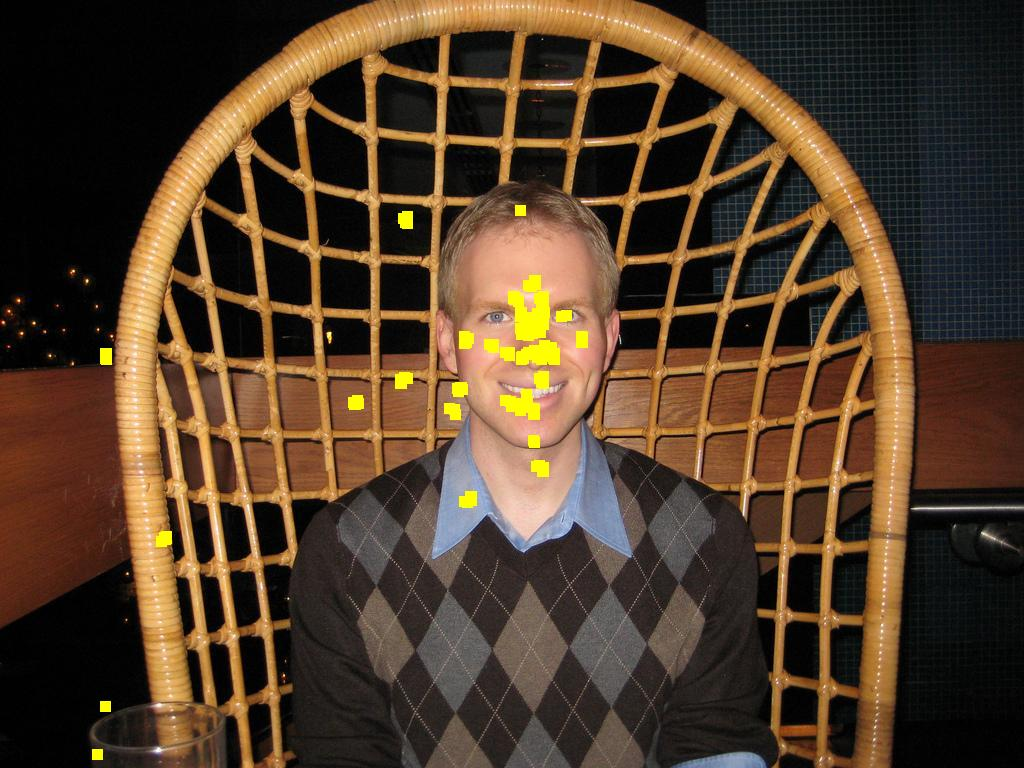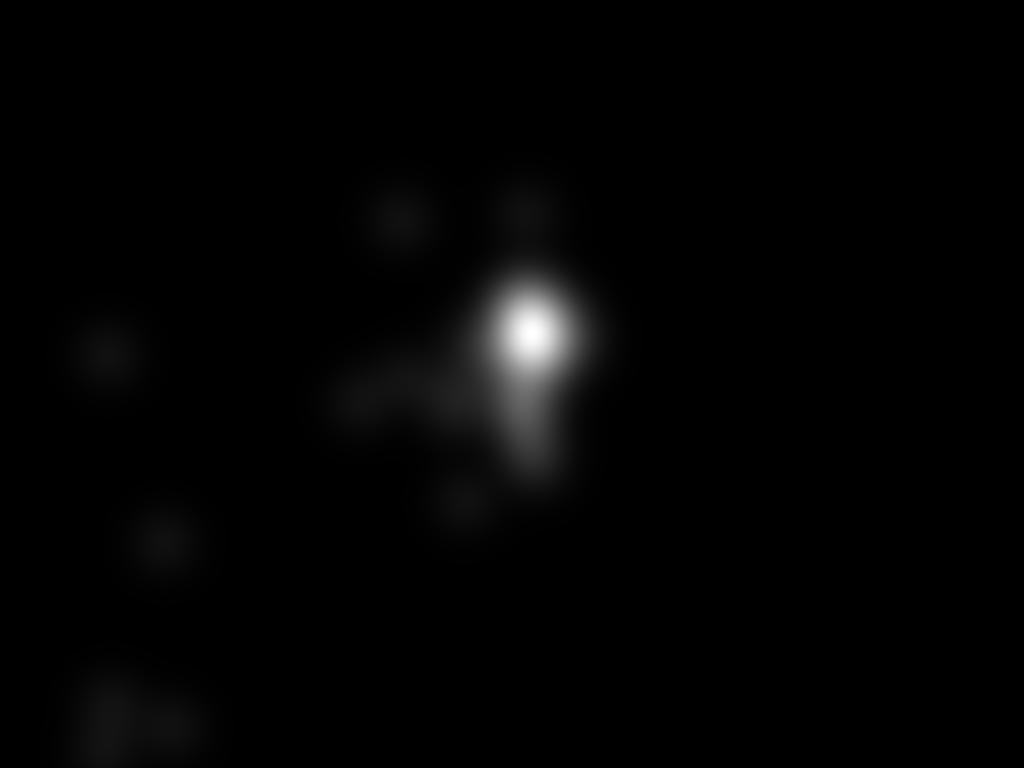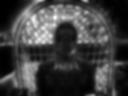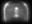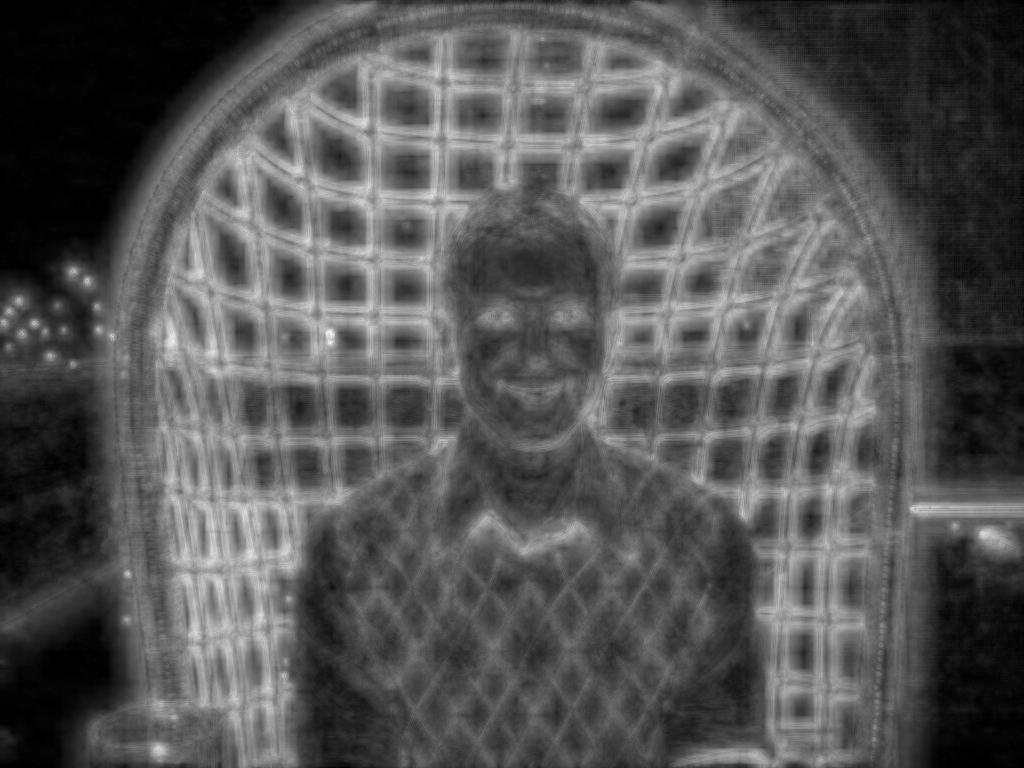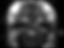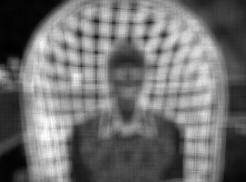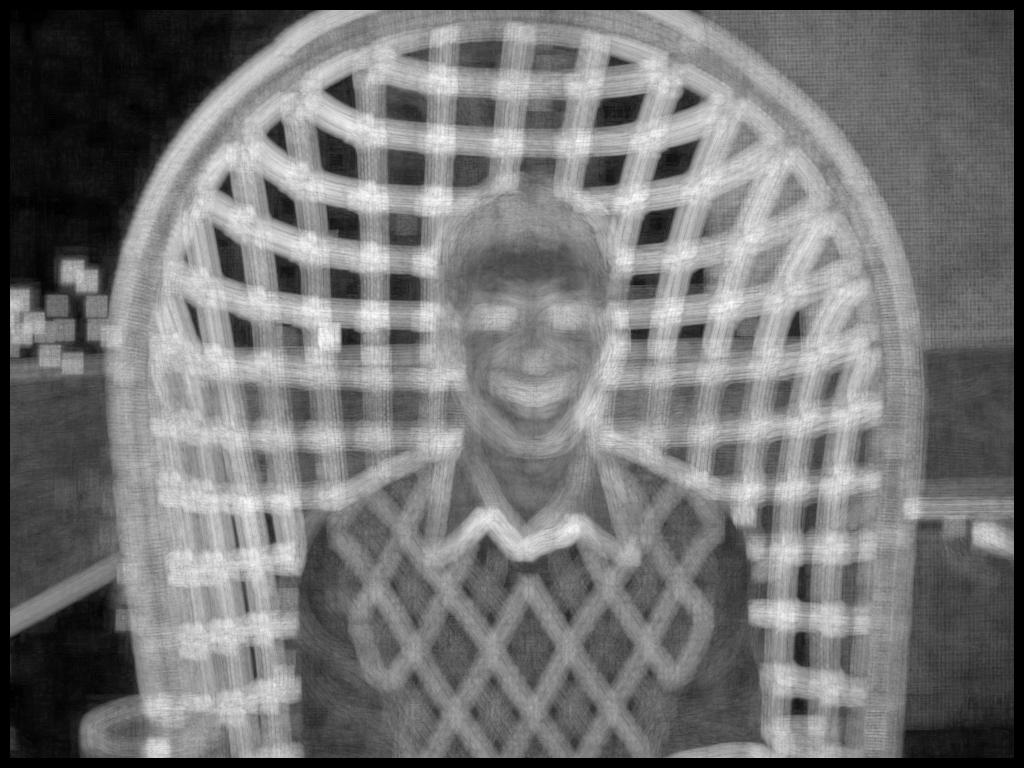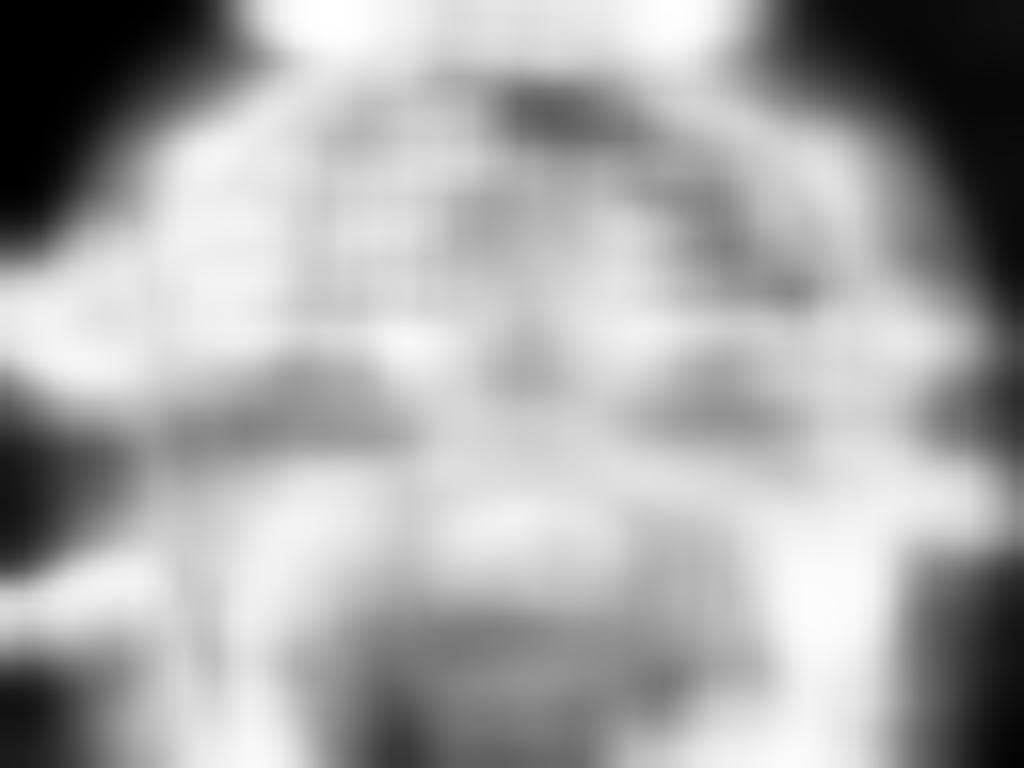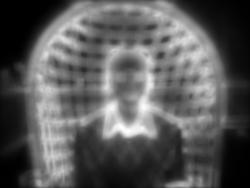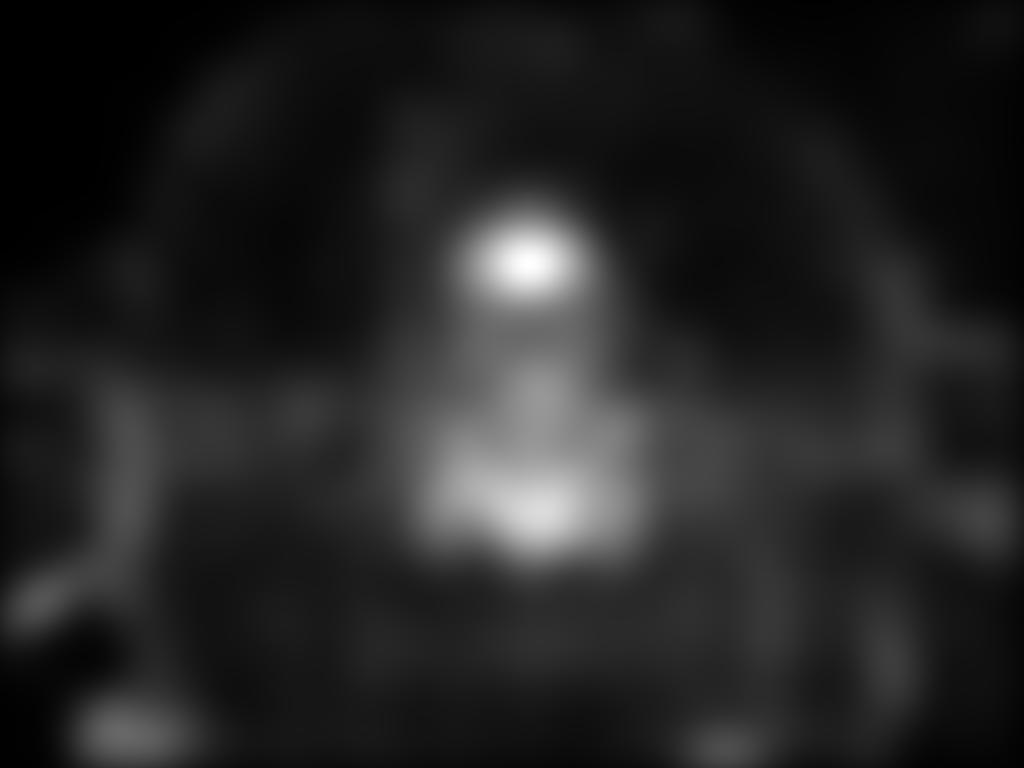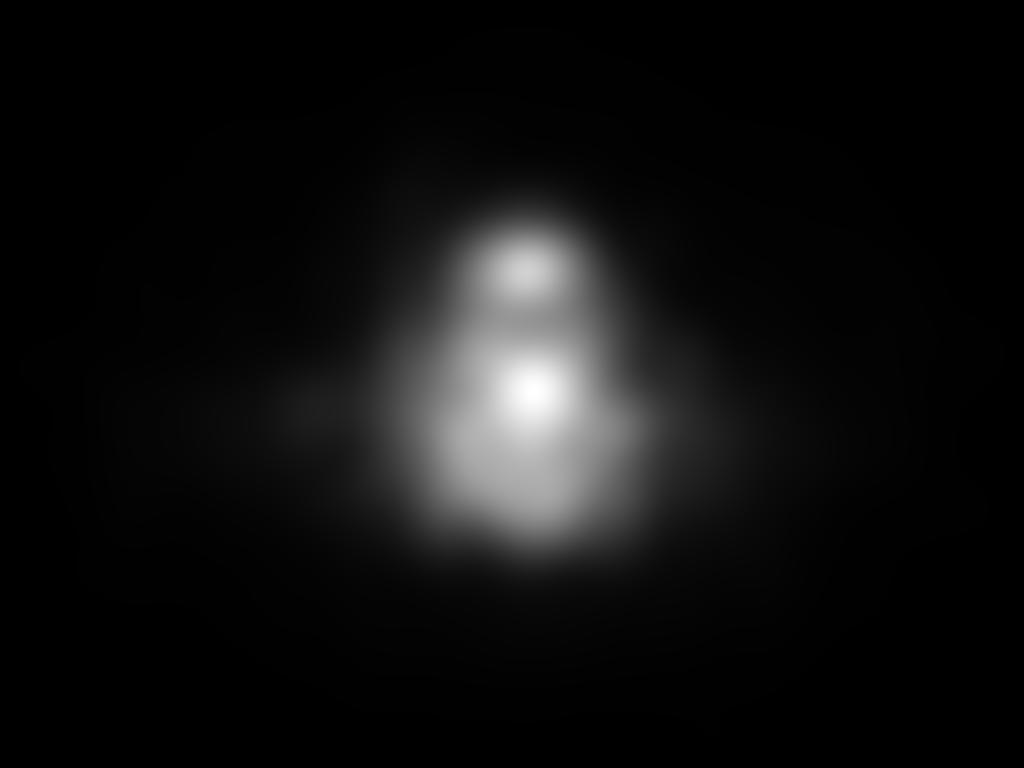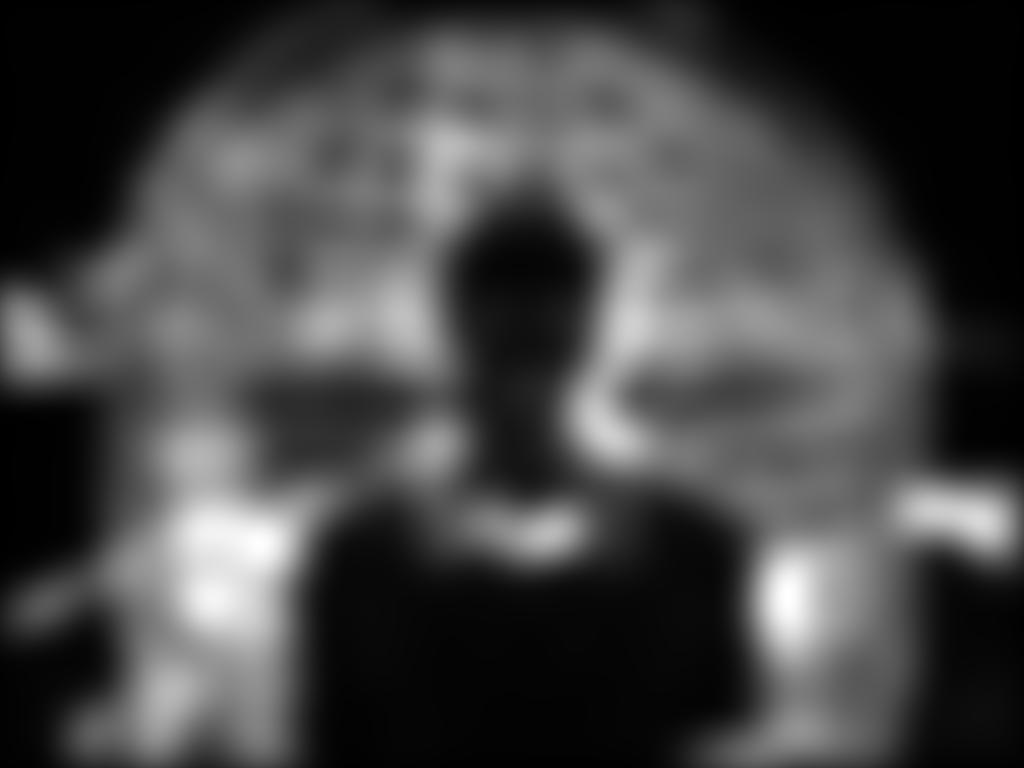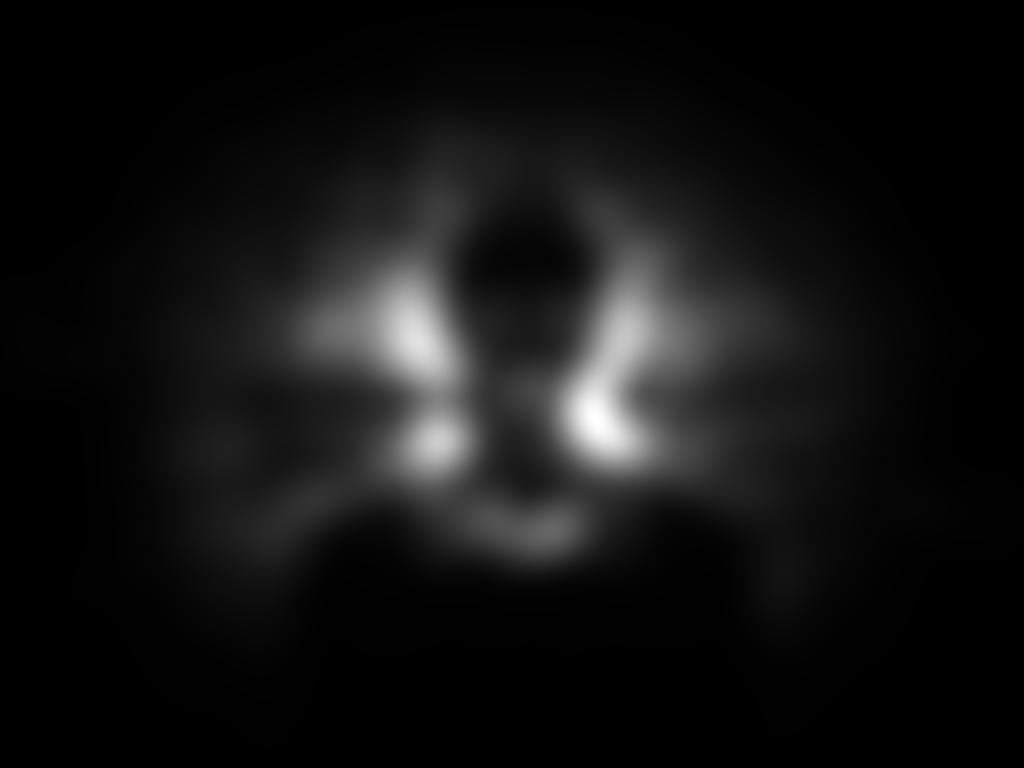Predicting human eye fixations

Toronto Data set

 Input Image Superimposed eye fixations Human density map Itti et al. (1998) Harel et al. (2007) Torralba et al. (2006) Hou and Zhang (2007) Zhang et al. (2008) Bruce and Tsotsos (2009) Seo and Milanfar (2009) Goferman et al. (2010) Our approach (Model 1) Our approach (Model 2) Our approach (Model 3) Our approach (Model 4)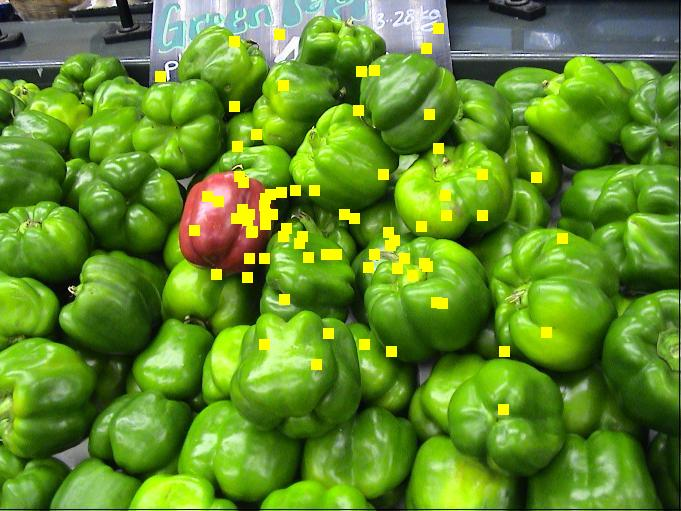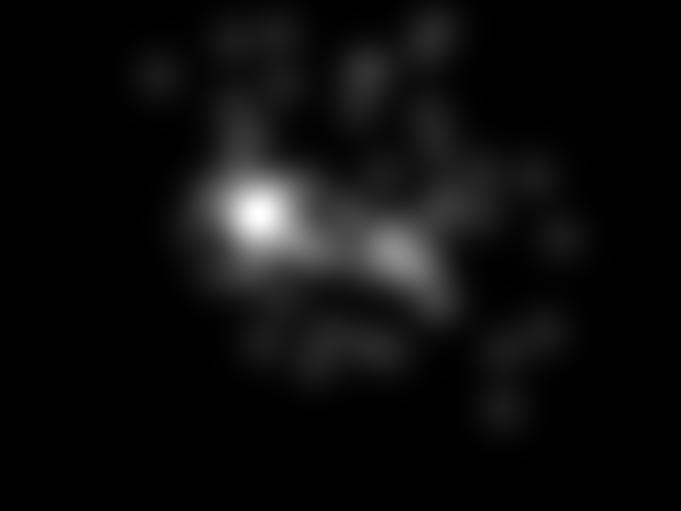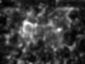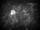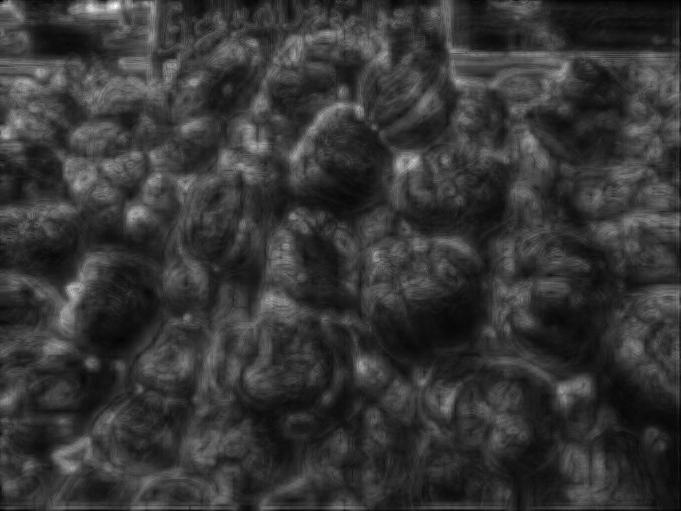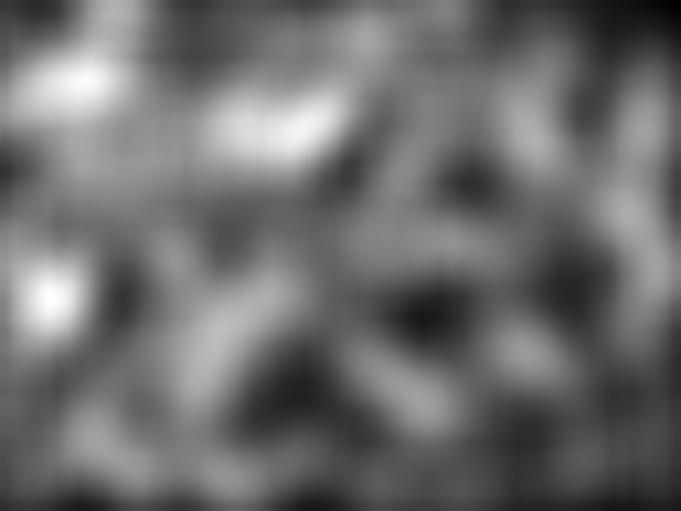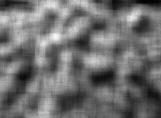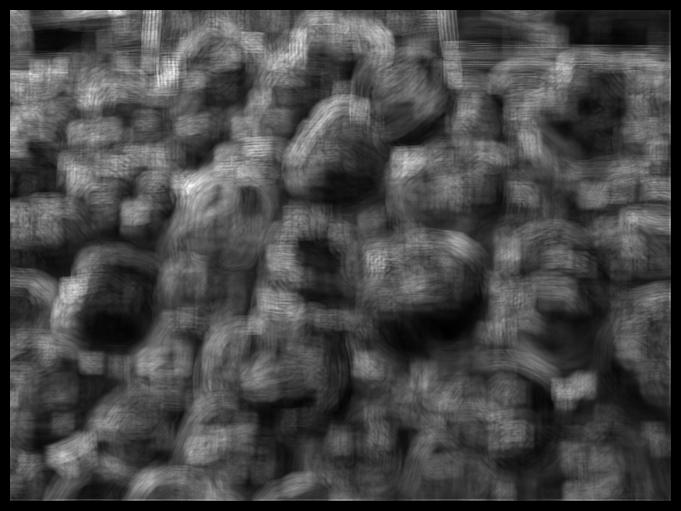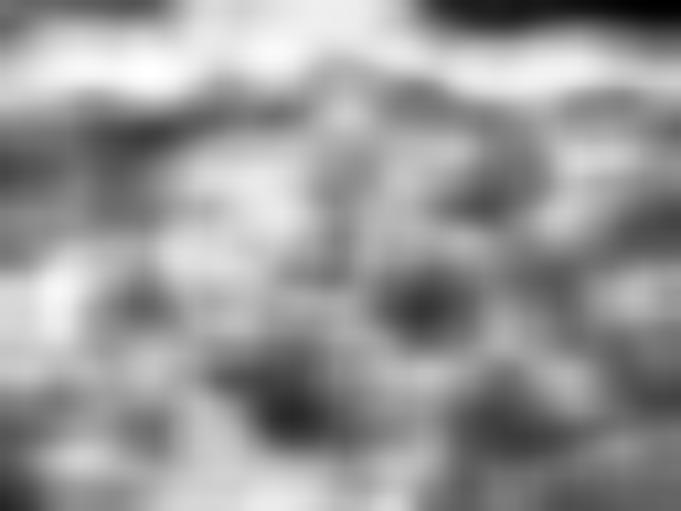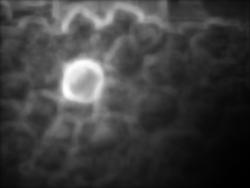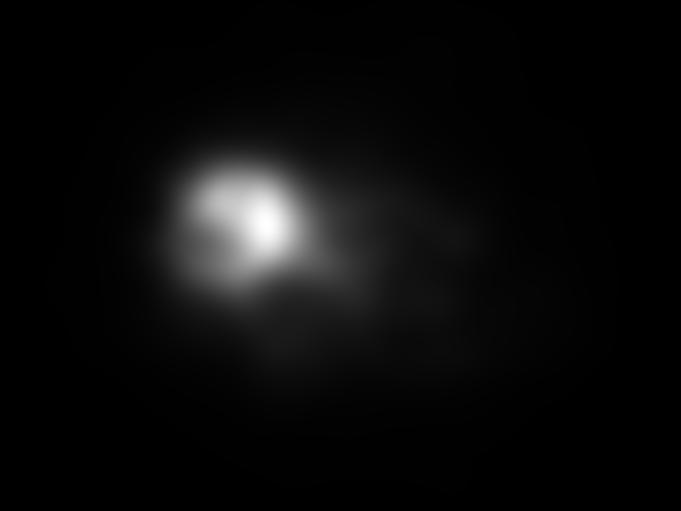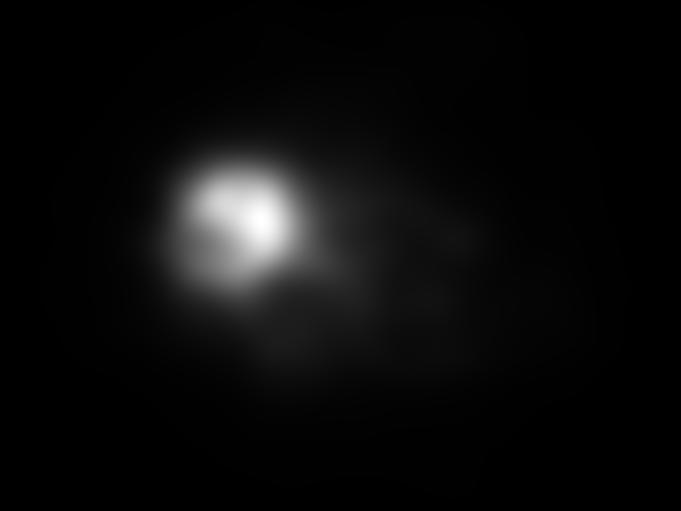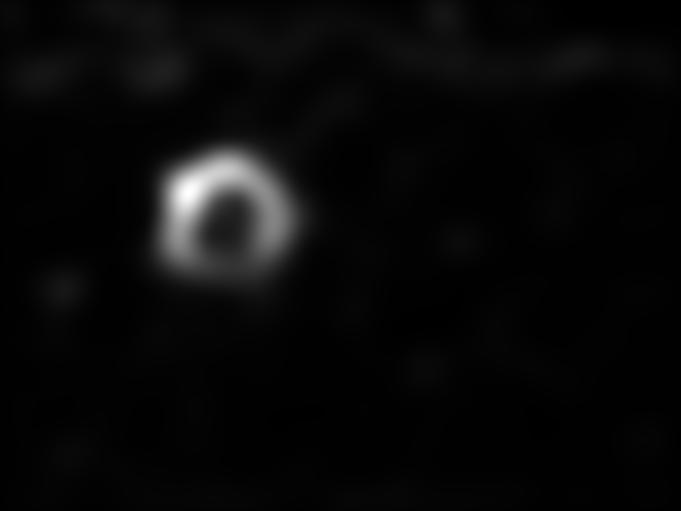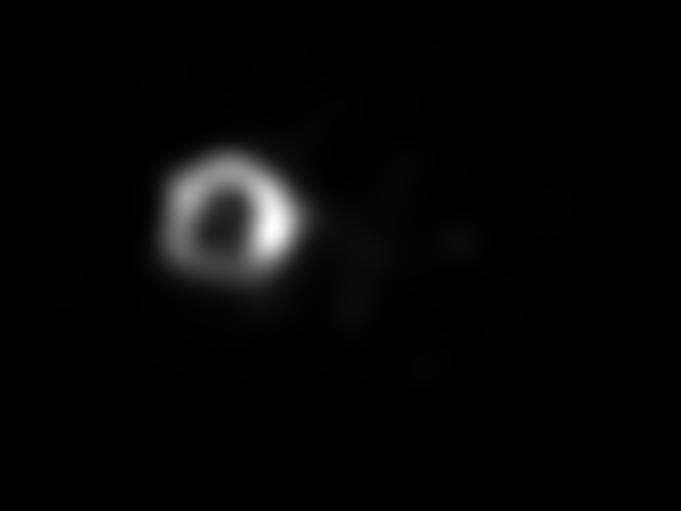Input Image Superimposed eye fixations Human density map Itti et al. (1998) Harel et al. (2007) Torralba et al. (2006) Hou and Zhang (2007) Zhang et al. (2008) Bruce and Tsotsos (2009) Seo and Milanfar (2009) Goferman et al. (2010) Our approach (Model 1) Our approach (Model 2) Our approach (Model 3) Our approach (Model 4)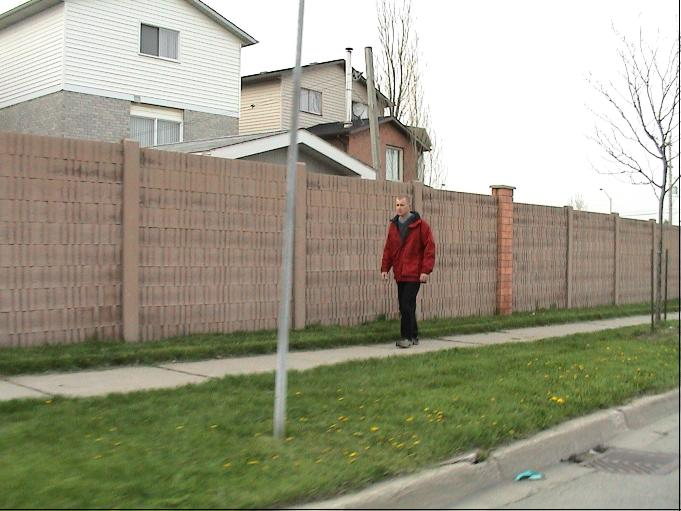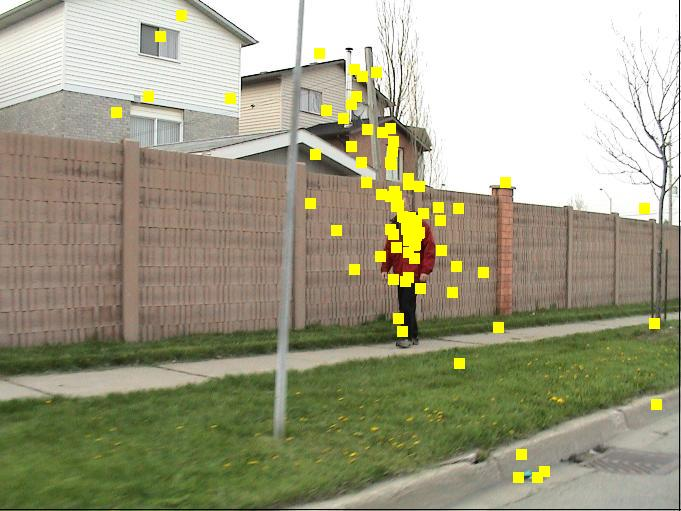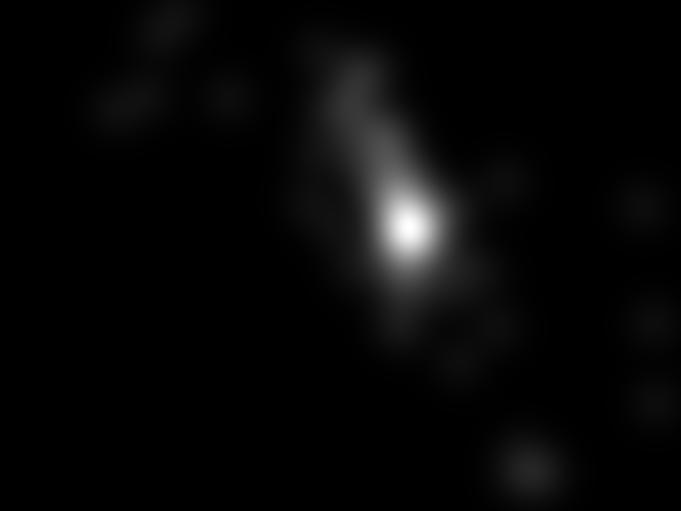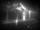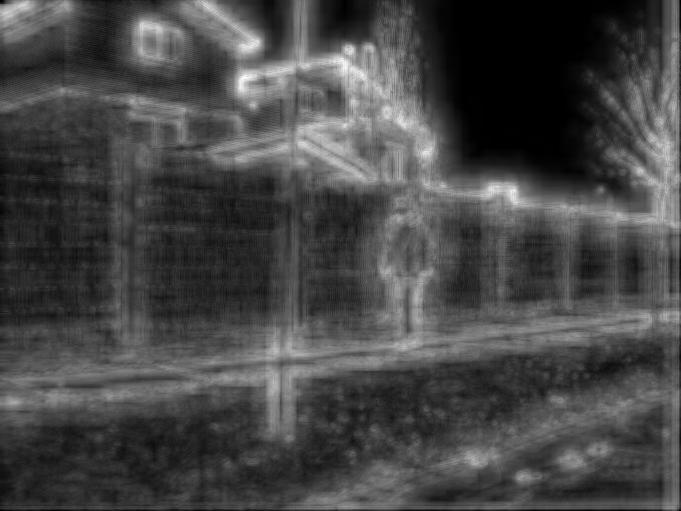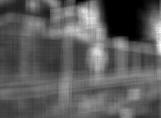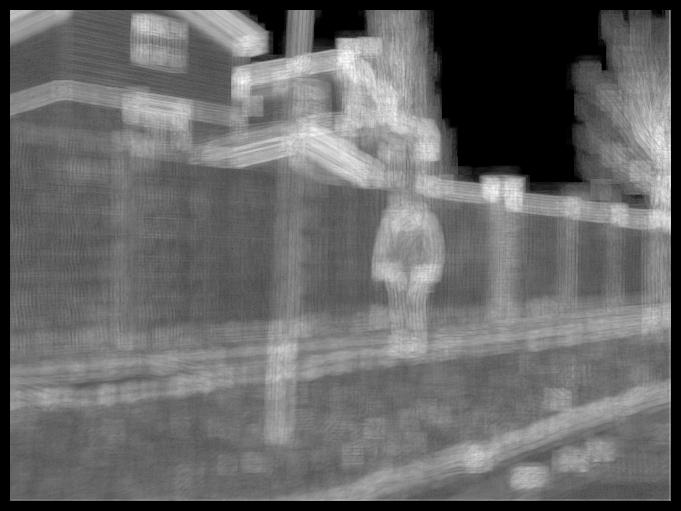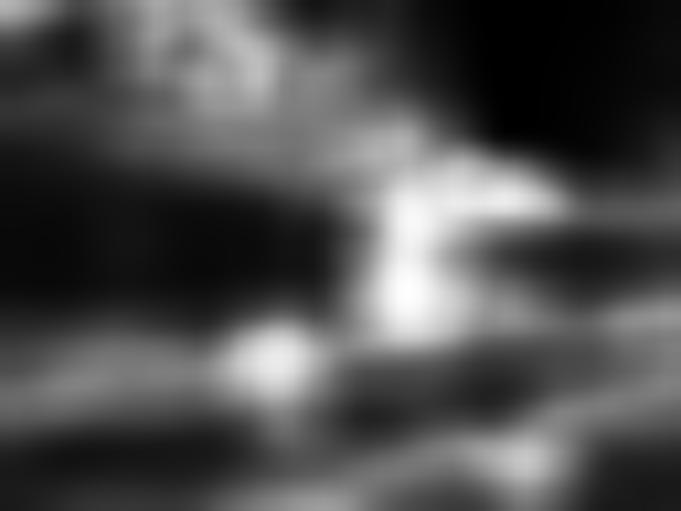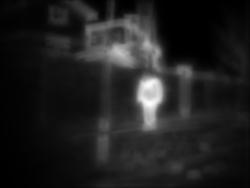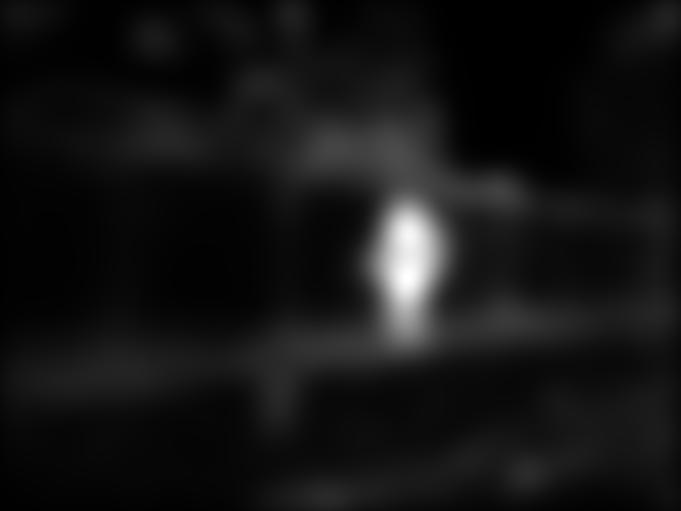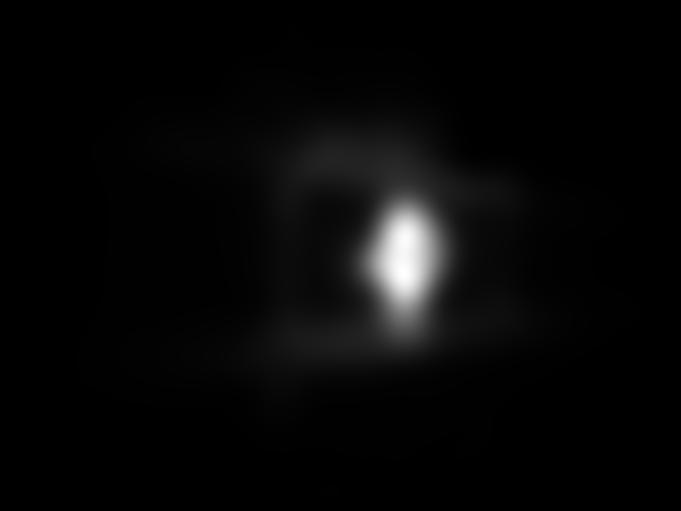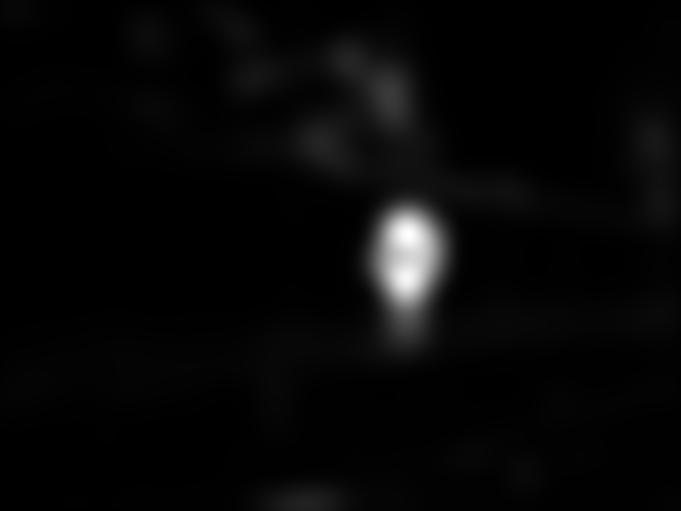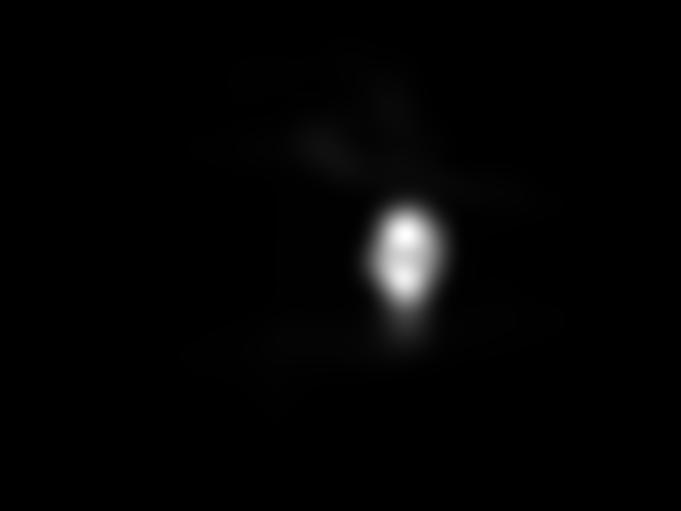Input Image Superimposed eye fixations Human density map Itti et al. (1998) Harel et al. (2007) Torralba et al. (2006) Hou and Zhang (2007) Zhang et al. (2008) Bruce and Tsotsos (2009) Seo and Milanfar (2009) Goferman et al. (2010) Our approach (Model 1) Our approach (Model 2) Our approach (Model 3) Our approach (Model 4)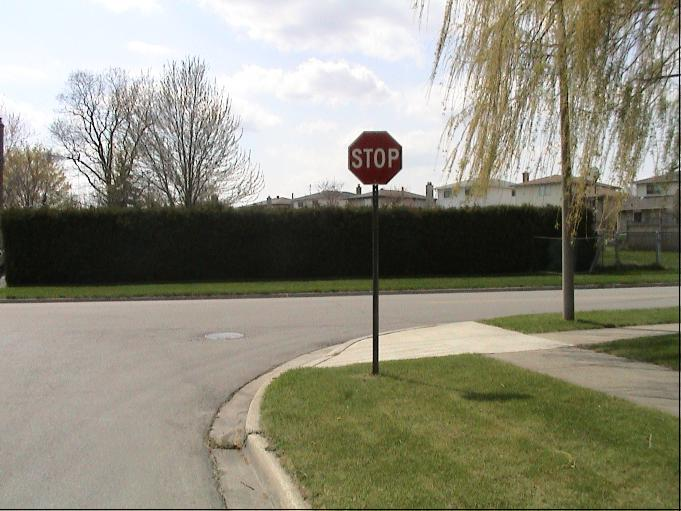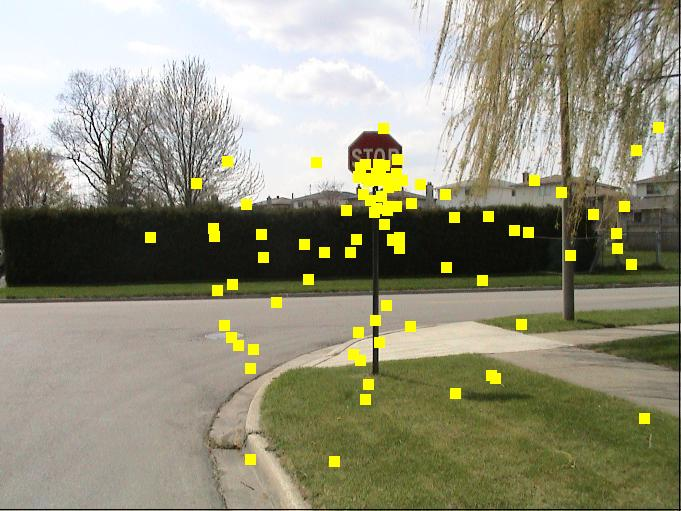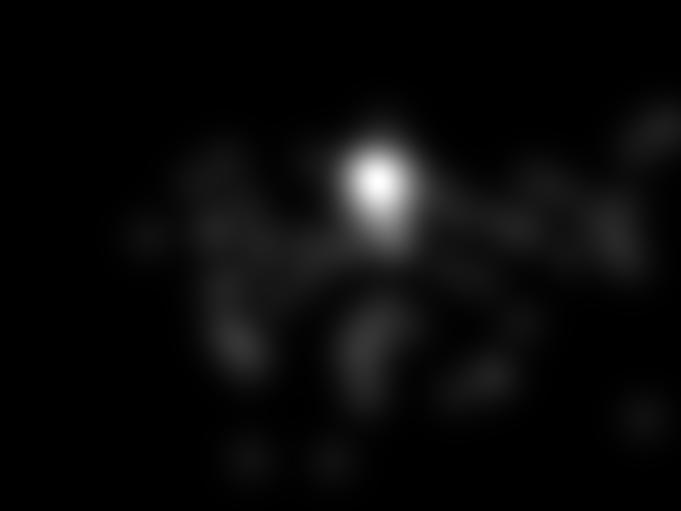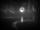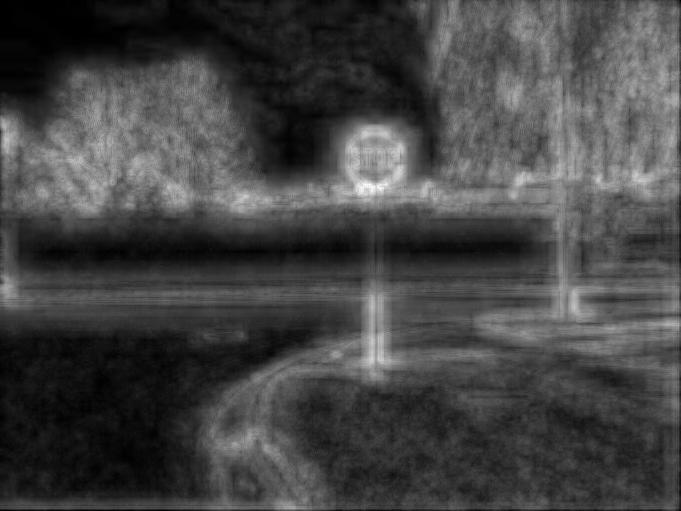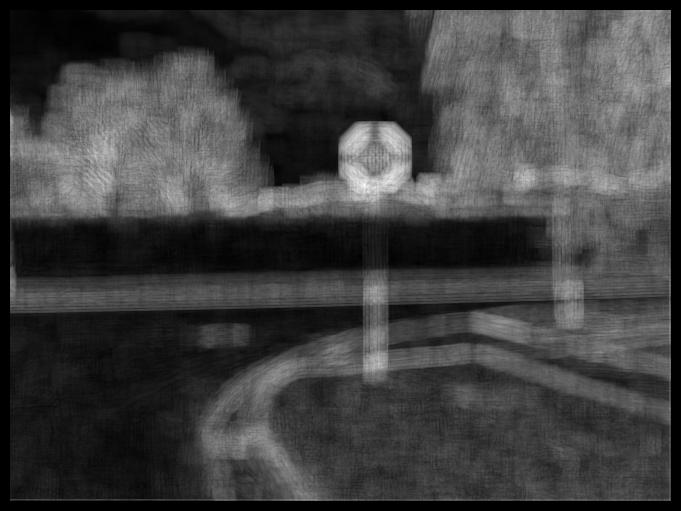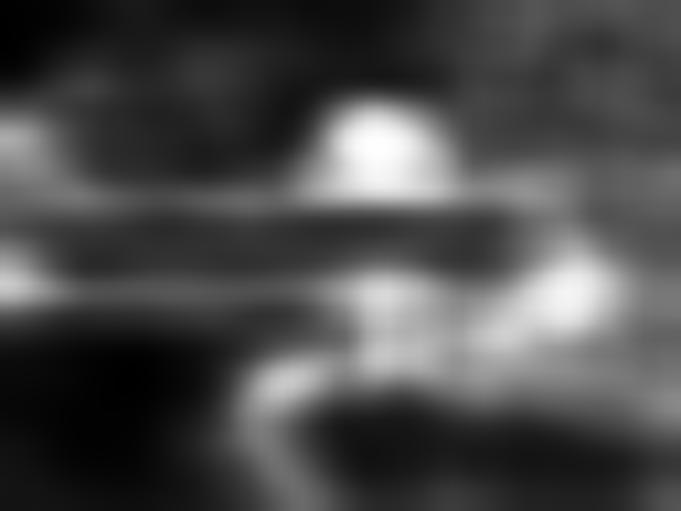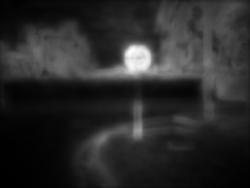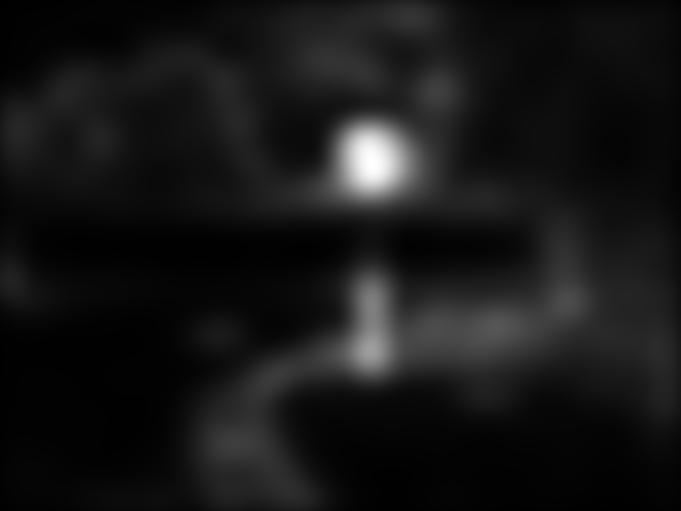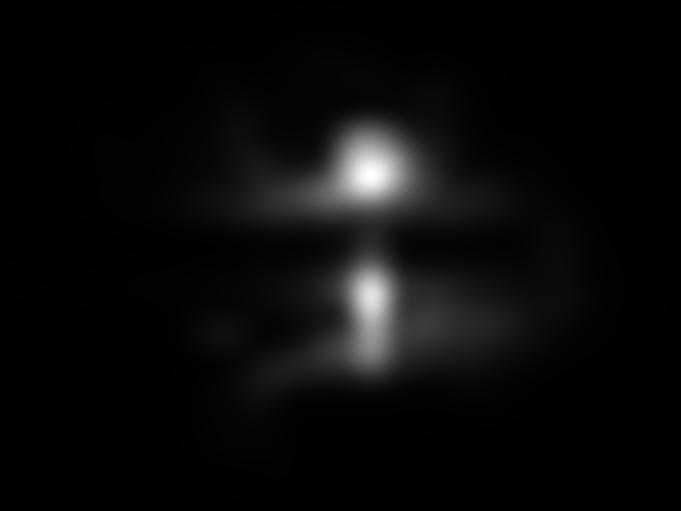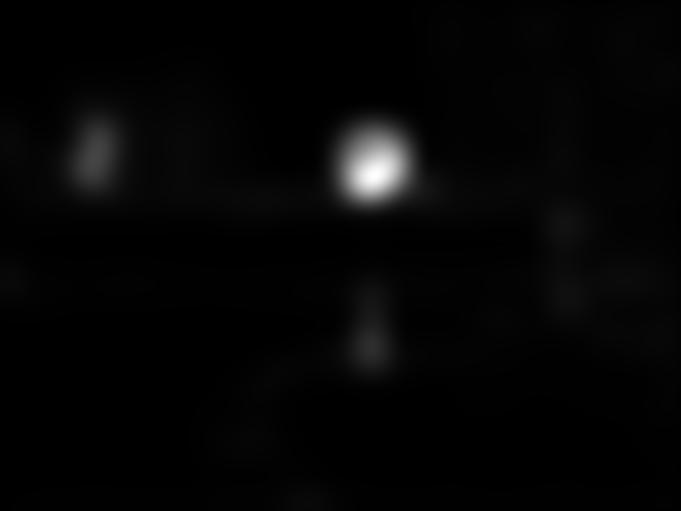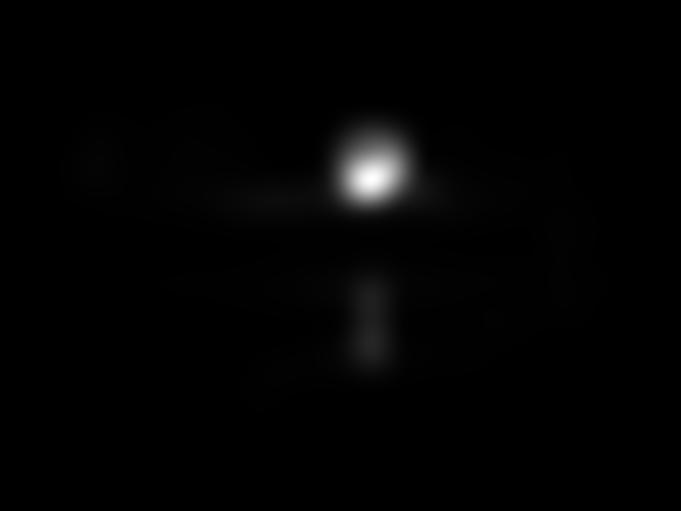MIT1003 Data set

 Input Image Superimposed eye fixations Human density map Itti et al. (1998) Harel et al. (2007) Torralba et al. (2006) Hou and Zhang (2007) Zhang et al. (2008) Bruce and Tsotsos (2009) Seo and Milanfar (2009) Goferman et al. (2010) Our approach (Model 1) Our approach (Model 2) Our approach (Model 3) Our approach (Model 4)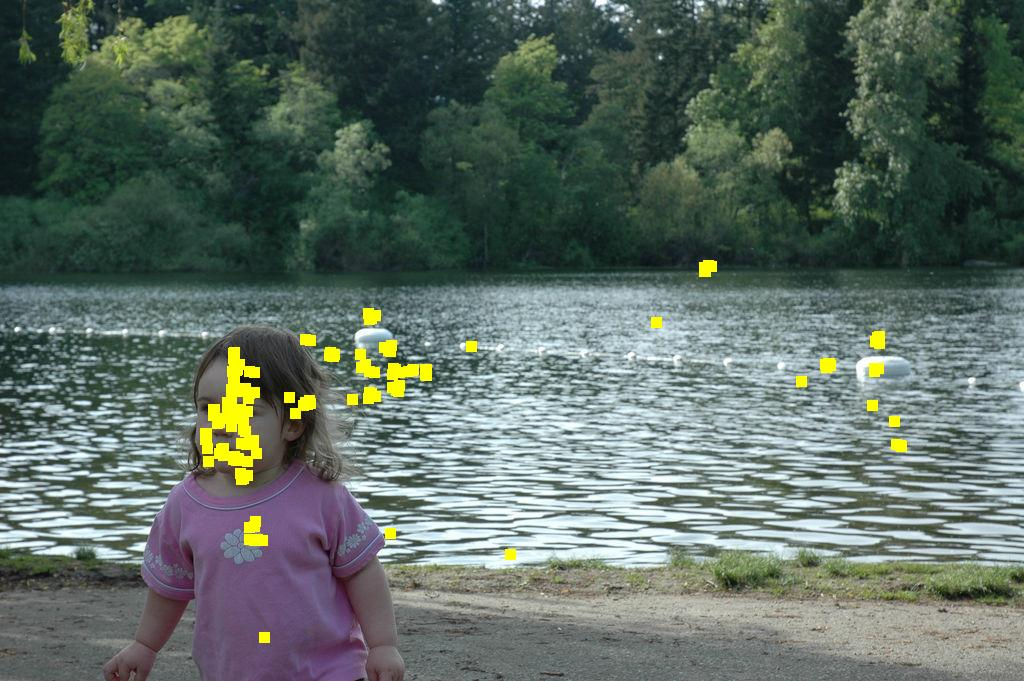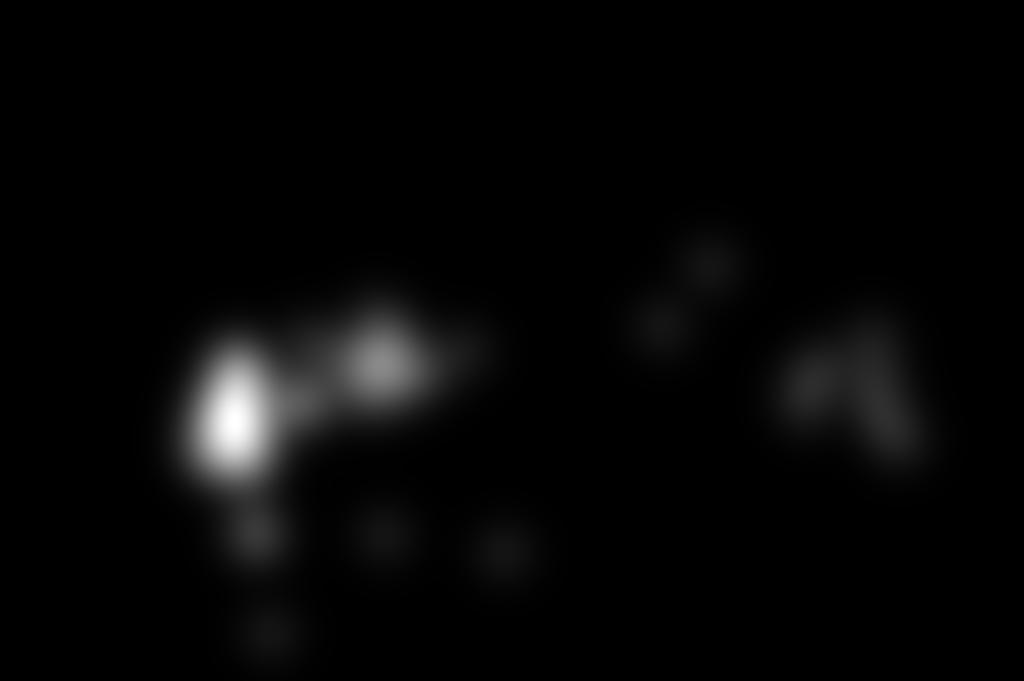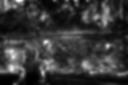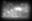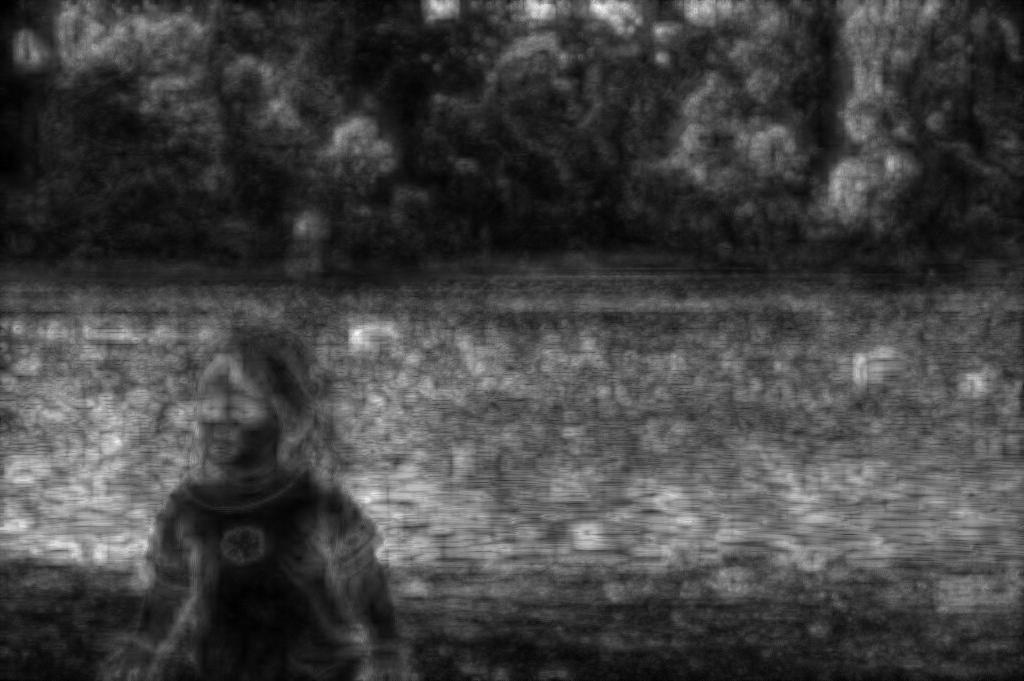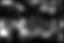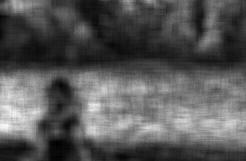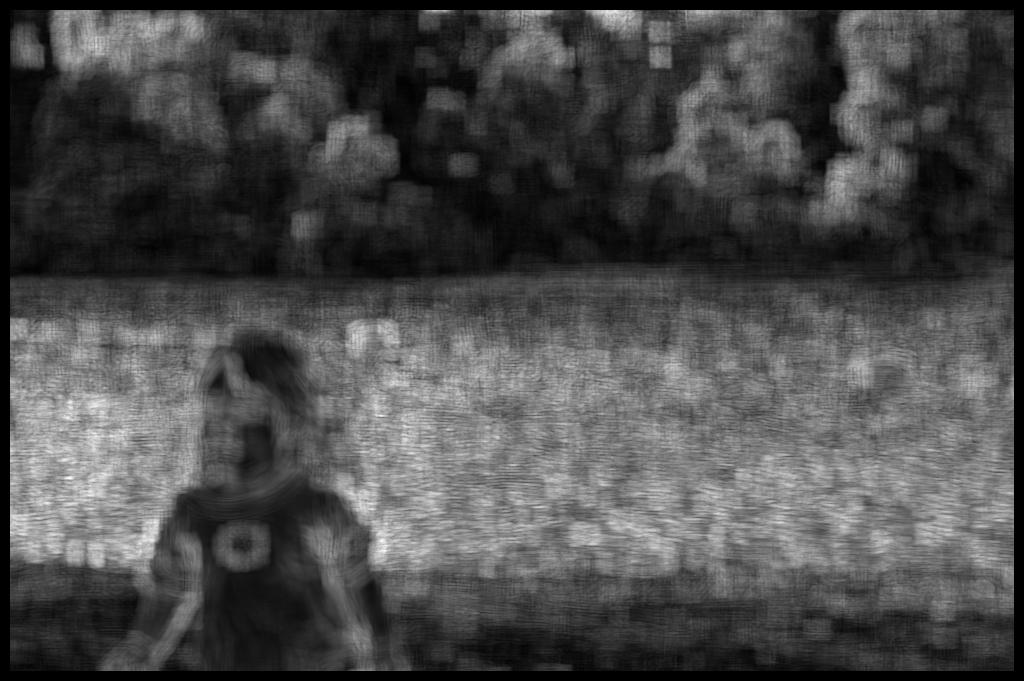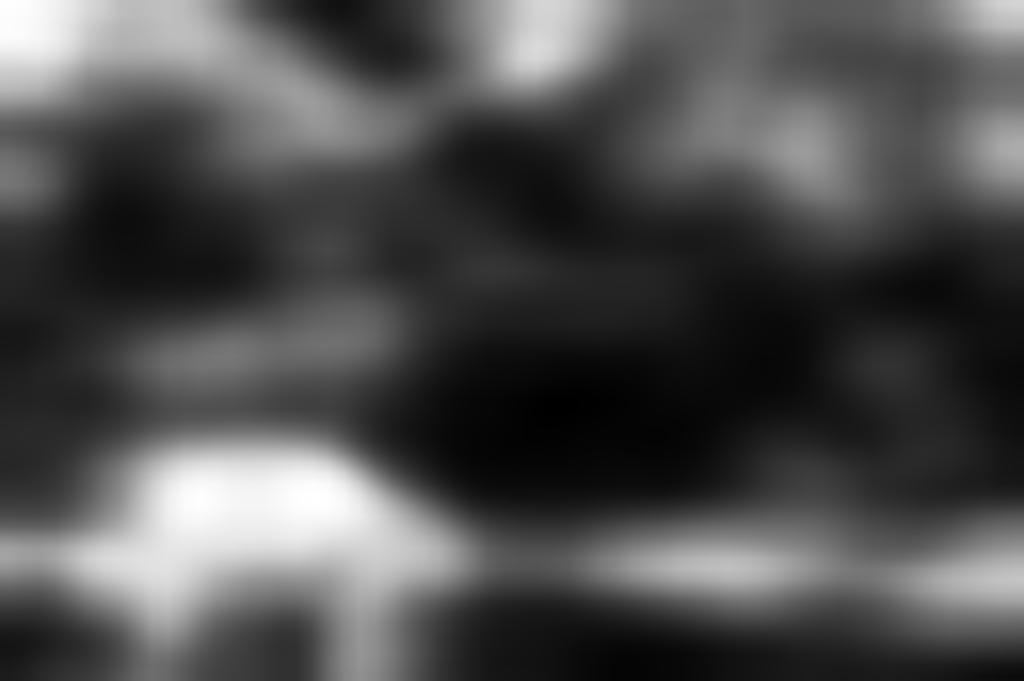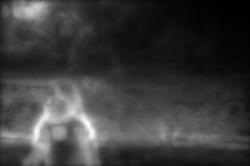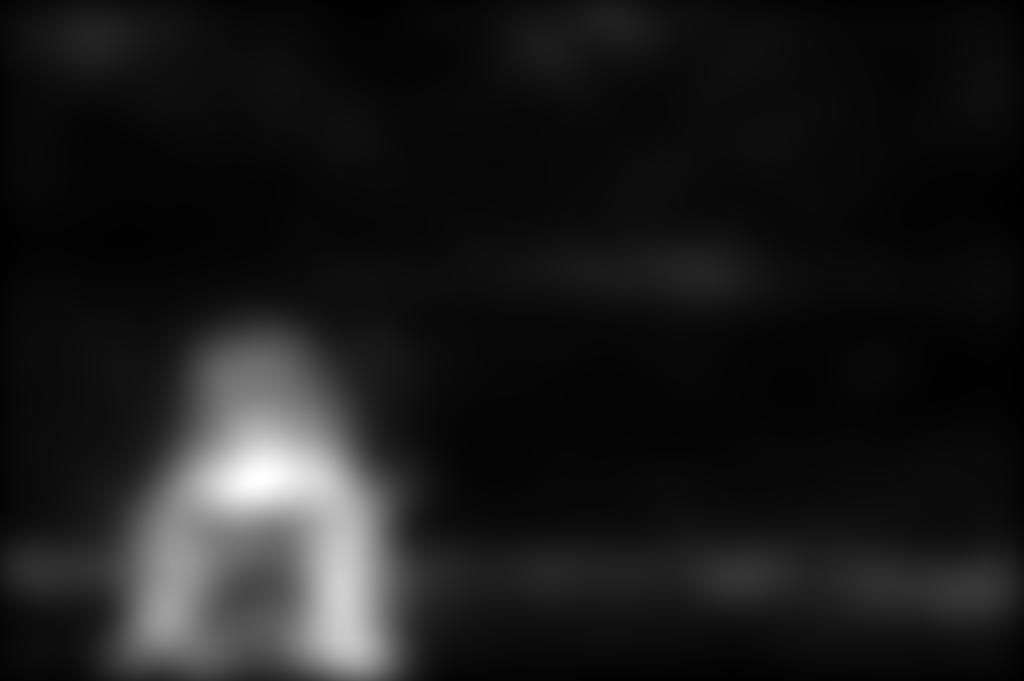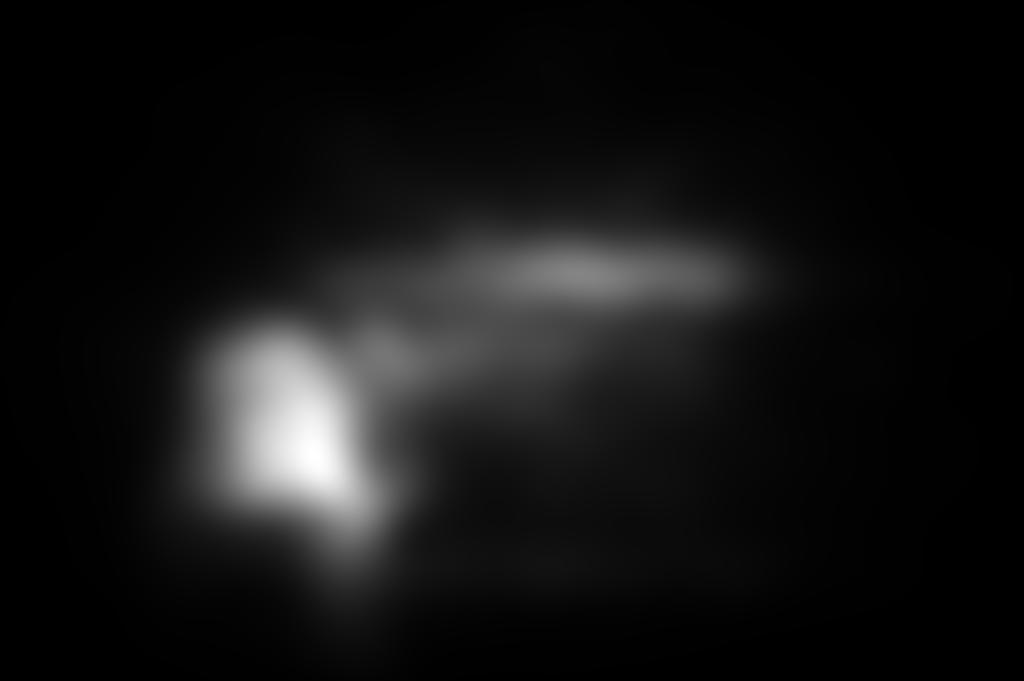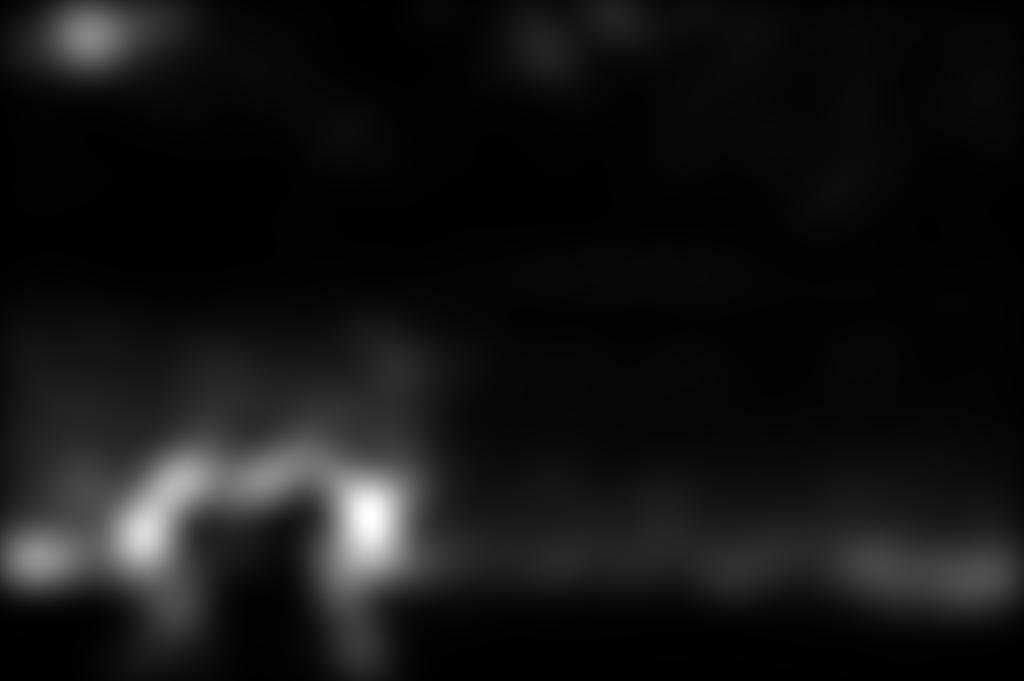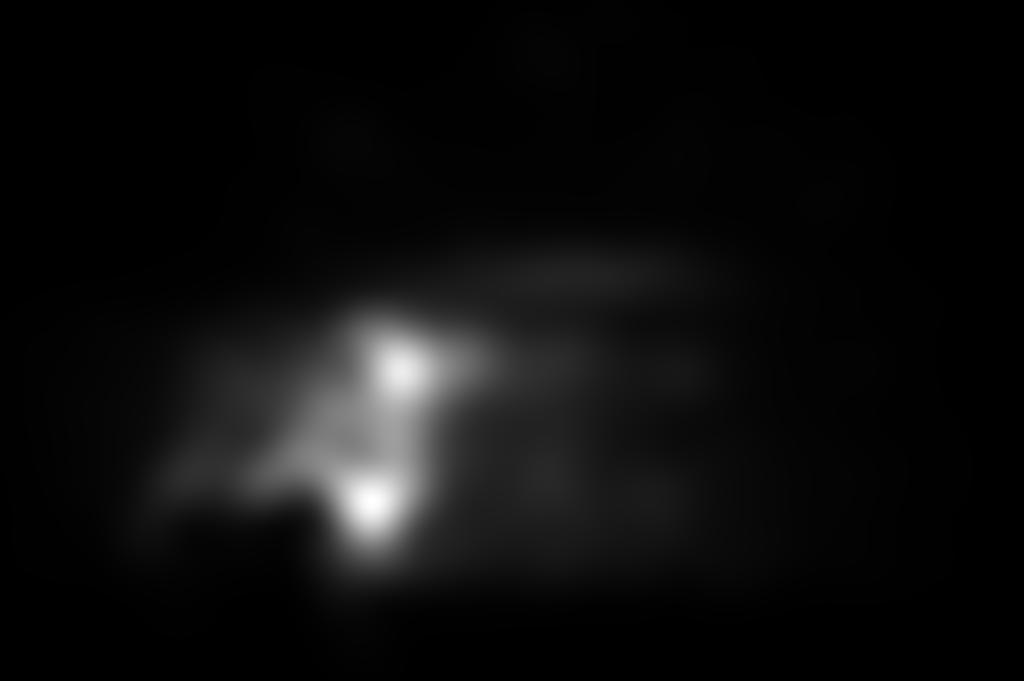Input Image Superimposed eye fixations Human density map Itti et al. (1998) Harel et al. (2007) Torralba et al. (2006) Hou and Zhang (2007) Zhang et al. (2008) Bruce and Tsotsos (2009) Seo and Milanfar (2009) Goferman et al. (2010) Our approach (Model 1) Our approach (Model 2) Our approach (Model 3) Our approach (Model 4)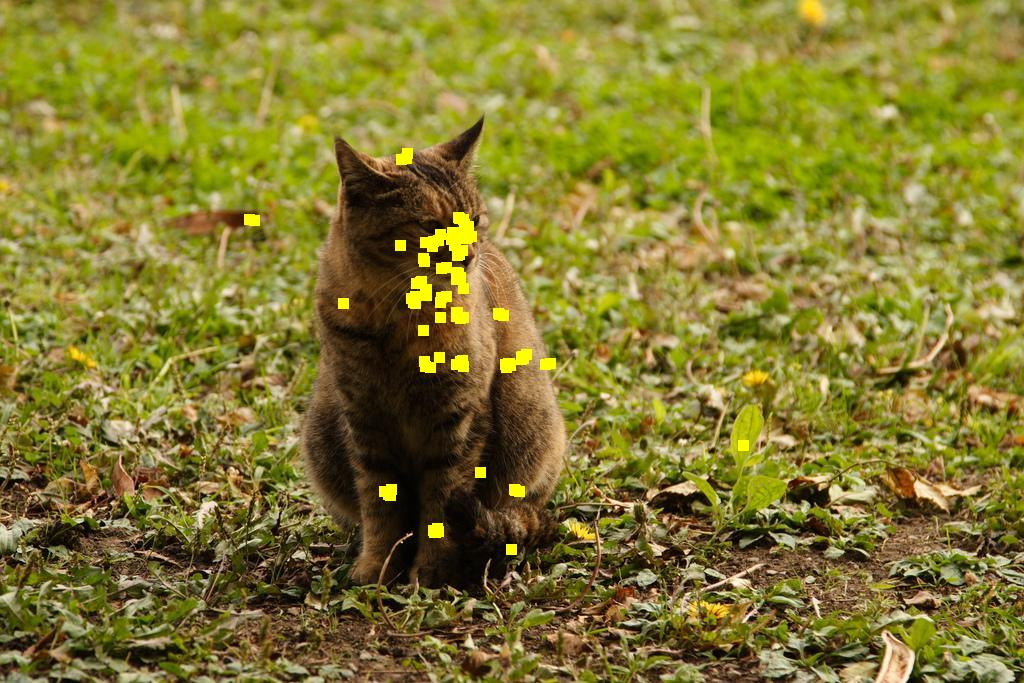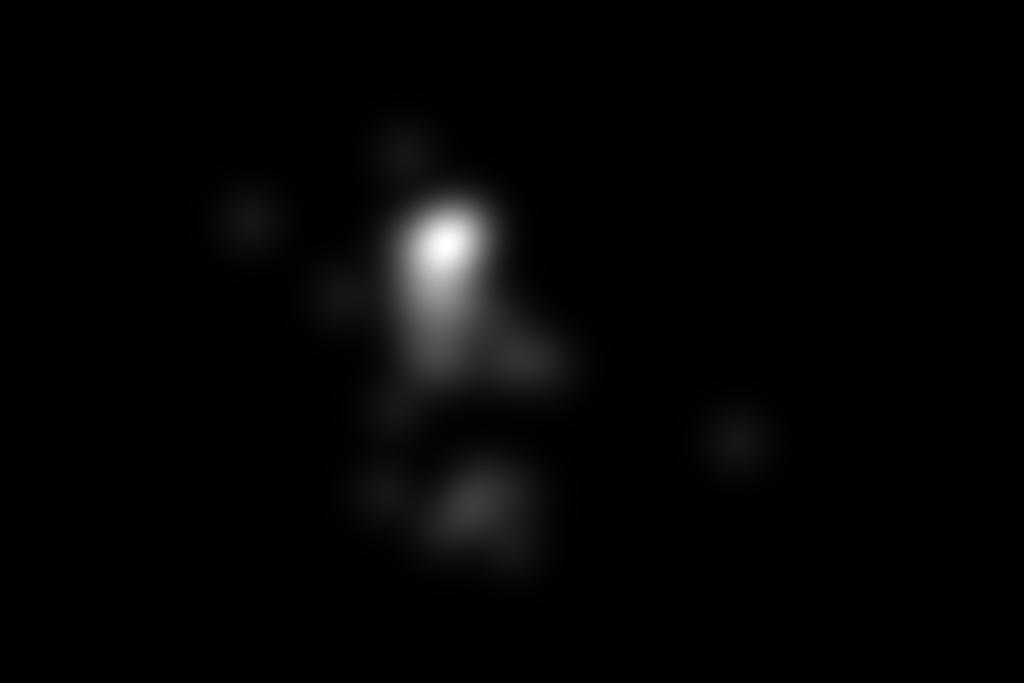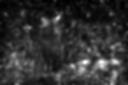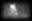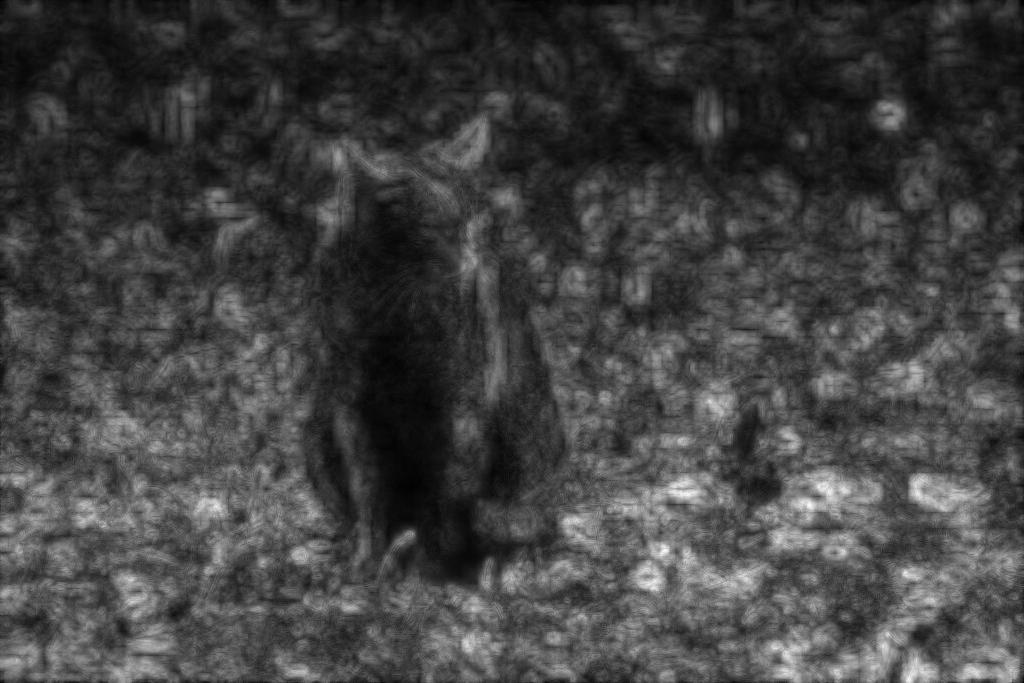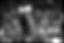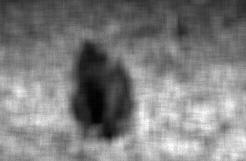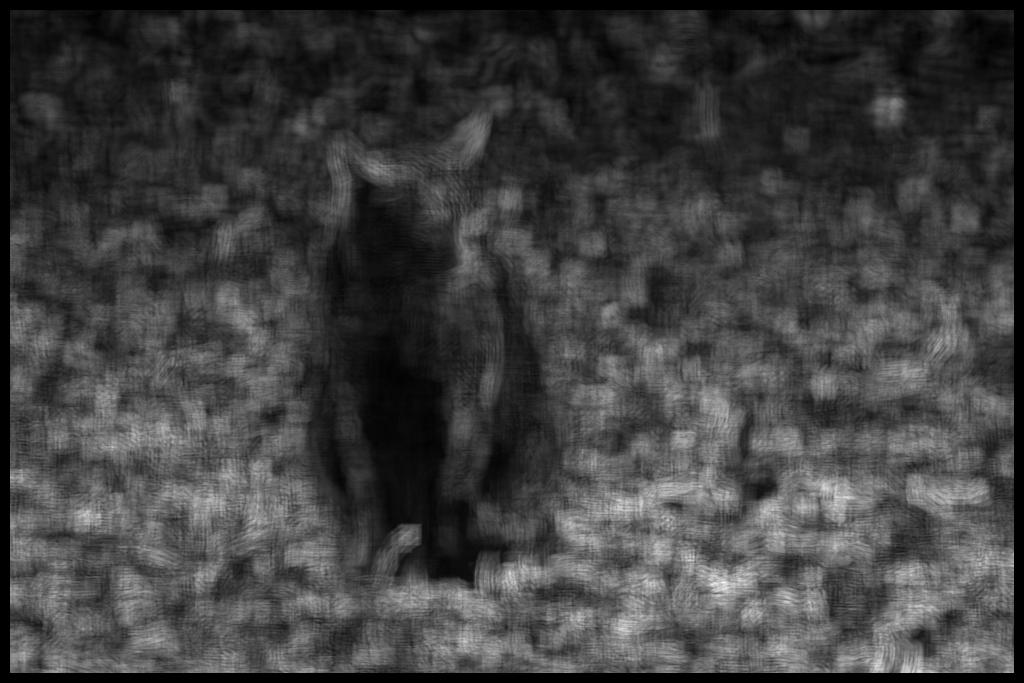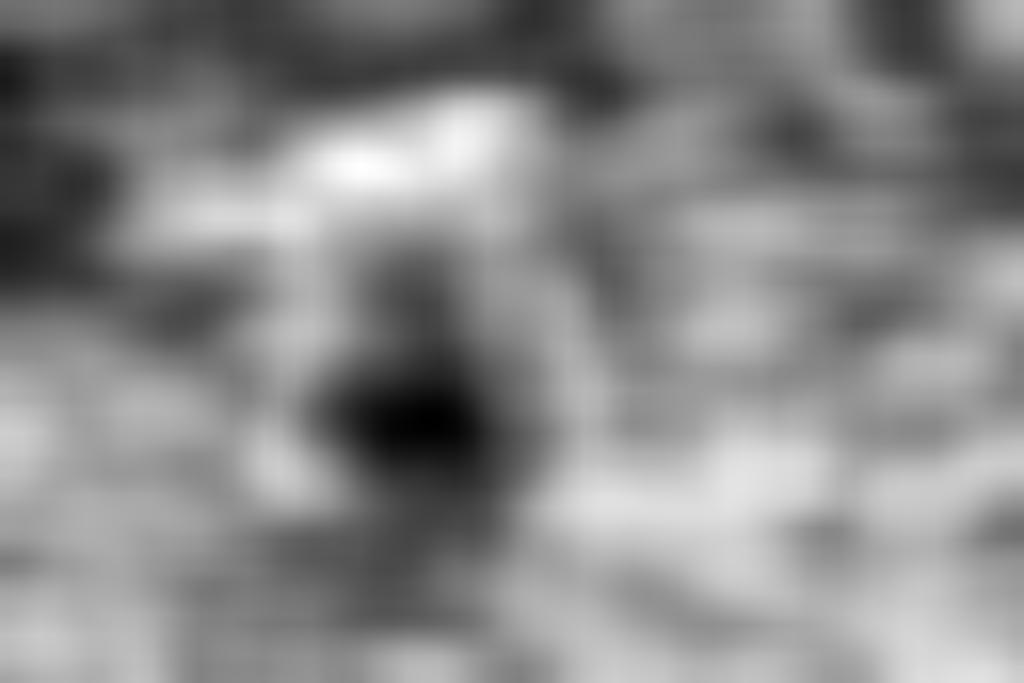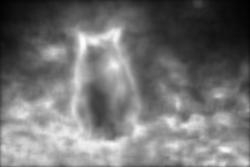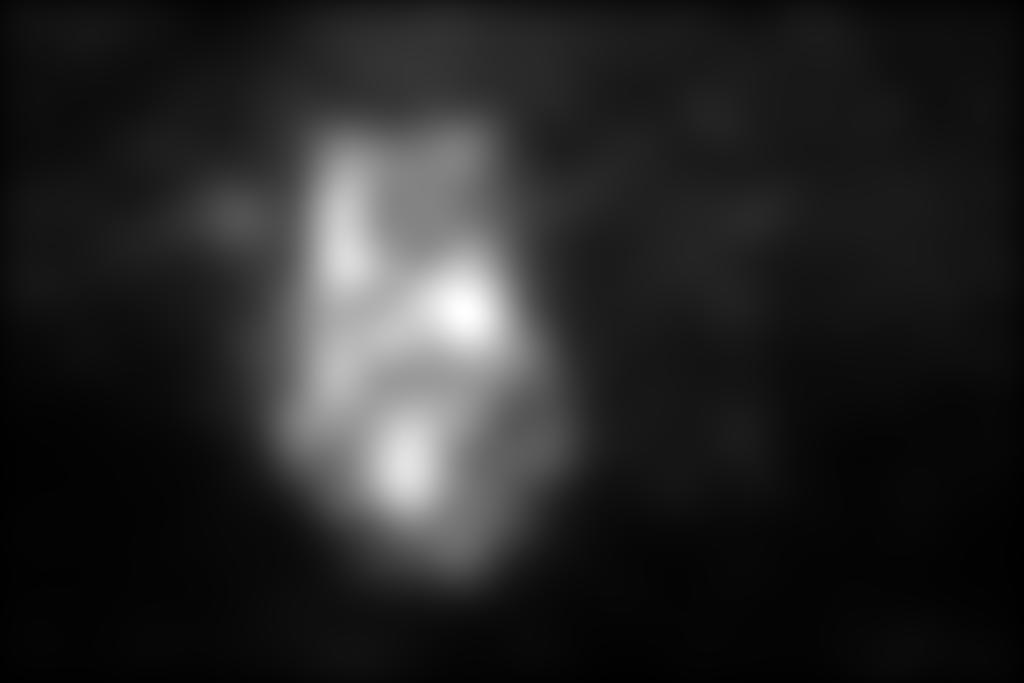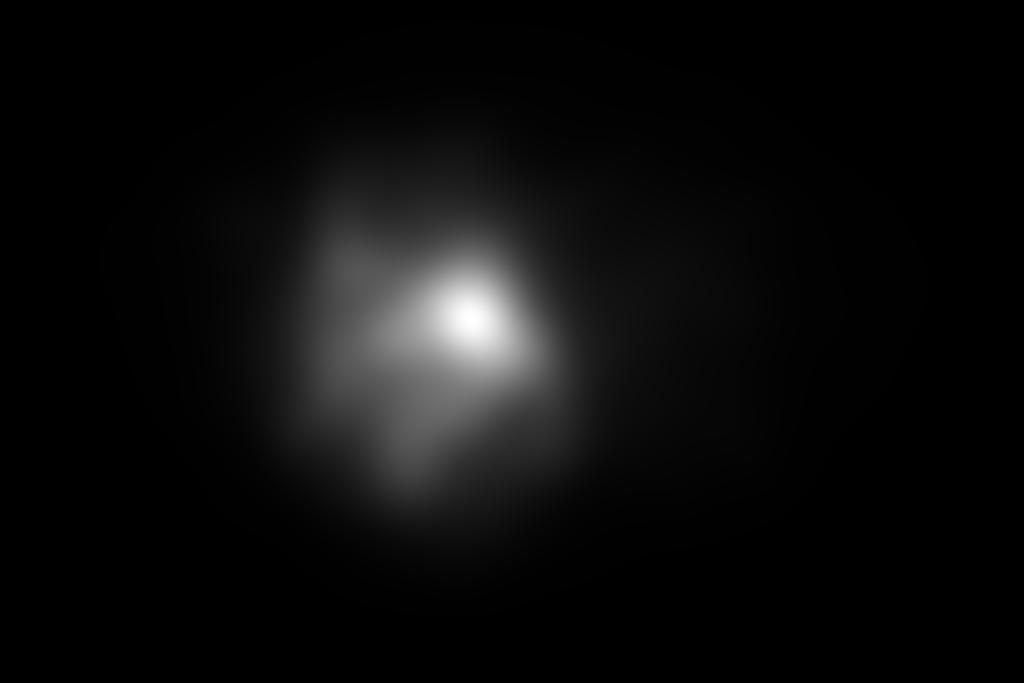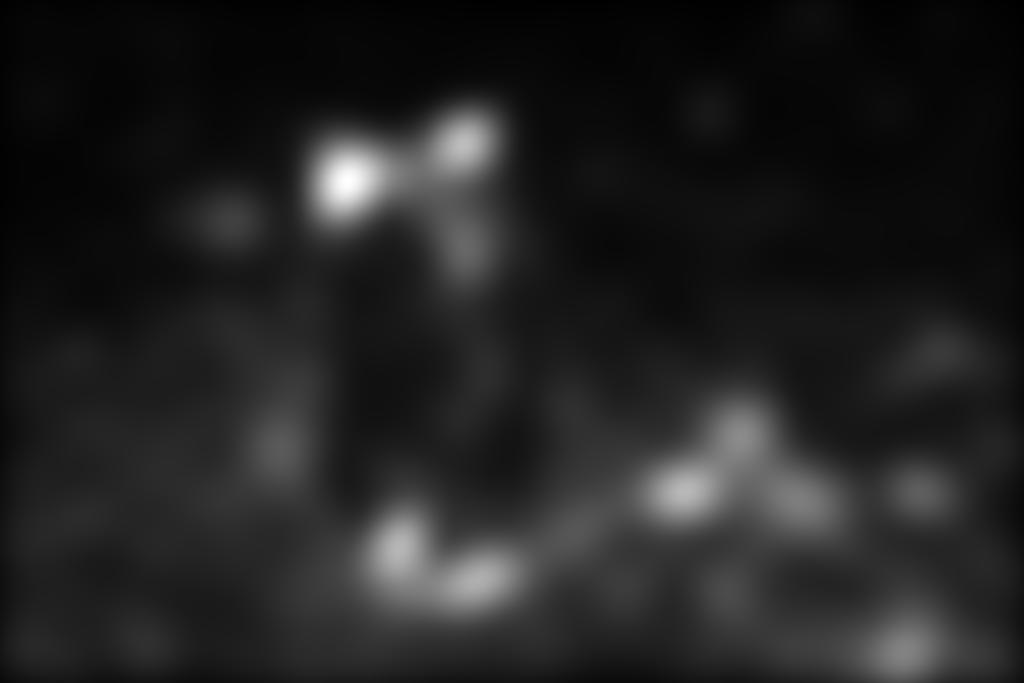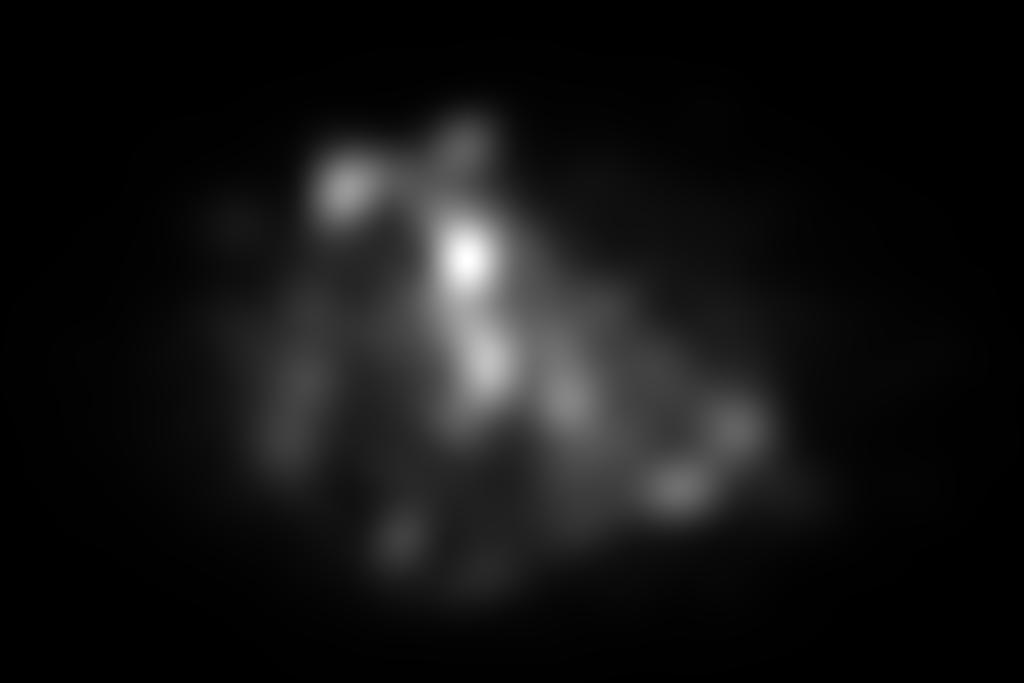Input Image Superimposed eye fixations Human density map Itti et al. (1998) Harel et al. (2007) Torralba et al. (2006) Hou and Zhang (2007) Zhang et al. (2008) Bruce and Tsotsos (2009) Seo and Milanfar (2009) Goferman et al. (2010) Our approach (Model 1) Our approach (Model 2) Our approach (Model 3) Our approach (Model 4)